MORE SITES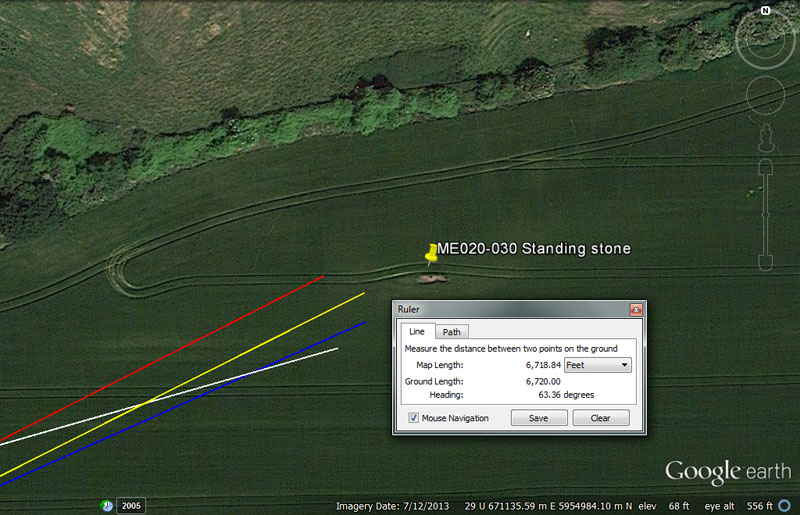A large standing stone marker could be found at this position. It probably doubled as a solar observatory and should be checked to see if, from this spot, there is an equinoctial or solstice rise or set, from or onto, a prominent geological feature to the ENE, East, ESE, WNW, West or WSW.

From Knowth = 19008’ @ 74.25-degrees.

Again, the value 19008' (3 X 6336') relates to measuring the equatorial circumference, under 3 different readings or navigational methods, in Egyptian Royal Cubits.

The degree angle is in homage to the 5940' mile, of which 742.5' would be 1/8th part. A length of 74250' would be 1/1760th of the 24750-mile equatorial circumference.

From Newgrange = 16666.6666’ @ 63-degrees.

Again, 16666.6666' is 1/3rd of 50000'.

The value 63 is a multi-use calculation number used in both lunar and navigational computations. The stadia increment from antiquity was 630'.

From Dowth Mound = 10368’ @ 66-degrees. Also, 10500'

This distance is 6000 Egyptian Royal Cubits of 20.736" each (1.728') found in the size of the paving slabs at the base of the Great Pyramid. Also the sum of 1036.8-miles would be 1/24th of the of the 24883.2-mile equatorial circumference.

This value was much used and very important anciently, as it described the speed at which the Earth spins in a day. Therefore 24883.2-miles ÷ 24-hours = 1036.8 MPH.

As a consequence to its importance it was encoded into volume measures and the Hebrew Jerusalem Cab for liquid volumes was 103.68 cubic inches. The Stirling Jug of Scotland for liquid volumes was also 103.68 cubic inches, obviously based upon a much earlier standard handed down from antiquity.

If this distance is read as 10500', then that's two miles of 5250' or 1000 Hebrew reeds of 10.5' each.

The angle code (66-degrees) is in homage to the chain @ 66' or furlong/furrowlong @ 660'.

From Dowth Henge = 6720’ @ 63.36-degrees.

This distance is, of course, an ancient Irish mile. Likewise, 6048' (1-minute of arc) is .9 of an Irish mile.

Again, the degree angle relates to measuring the equatorial circumference (3 methods) using Egyptian Royal Cubits (3 types).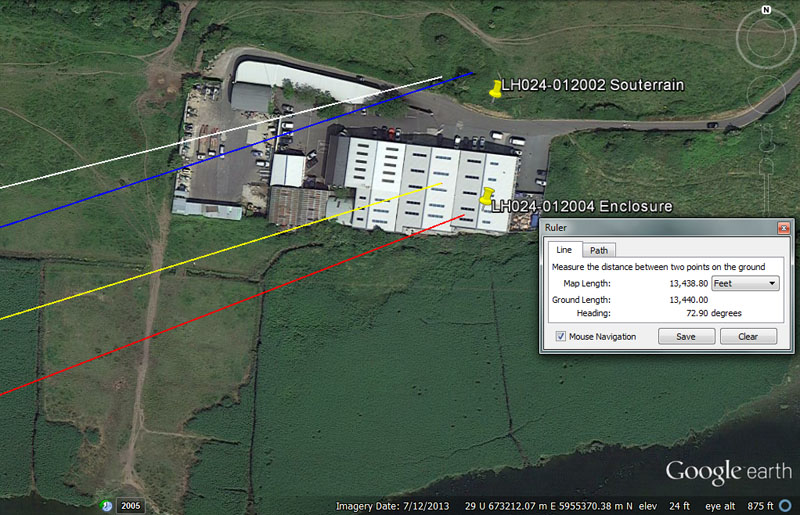Students issuing from the 4 main centres could find an enclosure, as well as souterrain accommodation here at Mell by navigating to the spot on the following distance and angle vectors:

From Knowth = 25920’ @ 256-degrees return.

This distance encodes the 25920-year duration of the Precession of the Equinoxes. The distance is also 15000 Egyptian Royal Cubits of 20.736" (20 & 92/125ths). It is also 1/5040th if the 24883.2-mile equatorial circumference. Likewise, the sum of 259.2-miles would be 1/96th of the equatorial circumference or 3.75-degrees of arc.

The degree angle is in homage to dividing up the 360-degree compass into 1/32nd parts and yet further sub-divisions of those parts.

From Newgrange = 23328’ @ 68.75-degrees.

This is 4.8 (of what much later became) Roman miles (using the 4860' reading). The 4861.1111 reading would produce 23333.3333'. The sum of 23328' would equate to 1/5600th of the equatorial circumference of the Earth under the 24883.2-mile (of 5250') reading.

The degree angle relates to the 24750-mile reading for the equatorial circumference, for which 68.75-miles = 1-degree of arc.

From Dowth Mound = 17187.5’ @ 72-degrees.

Again, the distance is navigational coding and 171.875-miles (171 & 7/8ths) would be 1/144th of the 24750-mile circumference or 2.5-degrees of arc. The distance of 17187.5' would be 10,000 Egyptian Royal cubits of 20.625" each.

The value 72 is multi-use. One example would be, in the 25920-year duration of the Precession of the Equinoxes, wherein the calendar day marking the equinox has to be adjusted by 1-day every 72-years (360 X 72 = 25920).

From Dowth Henge = 13440’ (2 Irish miles) @ 72.9-degrees.

The degree angle is both lunar and navigational coding and there would be 93 & 1/3rd intervals of 72.9-days in the 6804-day lunar nutation cycle. Also, 7.29-inches would be 1/8th of a Roman pace (using the 58.32" reading for the pace), etc.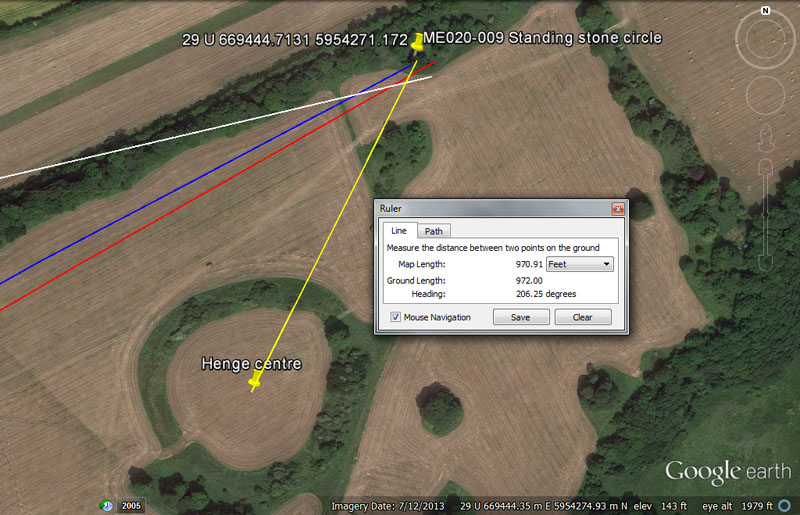Close to Dowth Henge is this, once magnificent, standing stone circle arrangement. Whereas the site is now substantially wrecked and the giant stones mostly missing, broken down and plundered for other uses (roading gravel, etc.), the position of the circle will be coded in length and angle relationships with the 4 main centres. We may never know the on-site codes, unless archaeologists can determine where each stone stood in relation to the centre hub stone. However, here are the apparent codes used by orienteers to locate the site when traveling from their dormitories.

From Knowth = 13125’ @ 76.8-degrees.

This distance is 2.5 miles of 5250' or 1/ 9953.28th of the equatorial circumference. It will be remembered that the intended length code from the centre of Dowth Henge to the centre of Newgrange was 9953.28' and that this value X 2.5 = 24883.2.

The angle of 76.8-degrees is navigational and the sum of 7.68-miles would be 1/9th of 1-degree of arc (69.12-miles) in the 24883.2-mile equatorial circumference. Alternatively, 76.8-miles would be 1/324th of the equatorial circumference.

From Newgrange = 10800’ @ 60 - 240-degrees.

This value is multi-use and can be applied to many types of calculations. there would be 108' X 56 in 1-minute of equatorial arc (6048'). There would be 240 X 108-years in the 25920-year Precessional cycle. There would be 63 intervals of 108-days in the 6804-day lunar nutation cycle, etc.

The 60 - 240-degree angles are self-explanatory and constitute 1/6th and 2/3rds of a 360-degree circuit, respectively.

From Dowth Mound =  4400’ @ 61.8034-degrees.

There would be 1.2 increments of 4400' in the 5280'-mile and 3.75 such increments in a league (16500').

The angle encodes the PHI reciprocal, which was used by assayer-mathematicians of the early and latter Mediterranean / European civilisations for squaring the circle such that very accurate volume tubs could be fabricated for the market places. The method used to generate this value was: 10-inches ÷ 1.6180339 = 6.18034-inches. To see why this was so important for maintaining ordered, functioning society, CLICK HERE. (then go midway down the page).

From Dowth Henge = 972’ @ 26.25 - 206.25-degrees return.

The sum of 97.2-miles would be 1/256th of the 24883.2-mile equatorial circumference.

The angle code is navigational and 2625' would be 1/2 a mile of 5250'.

The return angle (206.25-degrees) is in homage to the middle Egyptian Royal Cubit of 20.625" used in conjunction with miles of 5280' (3072 E.R.C).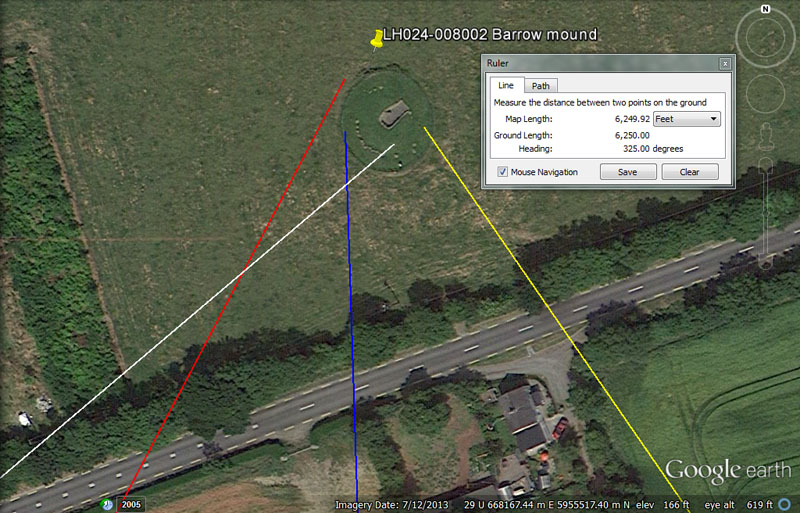This chambered mound offered comfortable conditions, out of the wind and rain, for orienteers needing a good nights sleep. It appears that centuries after the abandonment of the school someone might have decided it was a good place to stow uncle Eamon's corpse, so it's now registered as a barrow tomb. Whether or not any human remains were ever detected therein is unknown.

From Knowth = 11340’ @ 50-degrees.

This distance value is much used in lunar cycle computations and the 6804-day lunar nutation cycle is 6 intervals of 1134-days. Also, there would be 2.25 periods of 1134-days in the 2551.5-days of the lunisolar calendar. Greek historian, Herodotus was told by Egyptian priests that the Great Pyramid was 800' long. The priests were referring to an increment of 11.34" for lunar readings. Also, 3 side-lengths of the Great Pyramid equal 2268' (756' X 3) which equates to 1134' X 2. This 11340' distance is also 2.16-miles of 5250'.

The degree angle is self explanatory.

From Newgrange = 11000’ @ 28.35-degrees.

This distance is 2/3rds of a league (16500').

The degree angle is lunar. The Khafre Pyramid of Egypt had side lengths that were 15/16ths of those of the Great Pyramid (756'), thus 708.75'. One circumnavigation of the Khafre Pyramid (a pyramid of the moon) for all 4 sides was 2835'. If this value was read as 2835-days, then that would be 8 lunar years of 354.375-days each, to a tolerance about 12-minutes per year. If the 2835' distance around the pyramid were converted to inches, then the count would be (34020"), which is half of 68040". The lunar nutation cycle was anciently configured to be 6804-days or 283.5-days X 24.

From Dowth Mound = 6336’ @ 358.4-degrees.

Again, the key value for determining the various equatorial circumferences under 3-methods, according to readings in 3 slightly different Egyptian Royal Cubits. As stated, 6336' is 1.2-miles of 5280'.

If the 24883.2-mile (of 5250' each) equatorial circumference were read as miles of 4860' each then 130636800' ÷ 4860' = 26880-miles (Roman). Under this reading 1/75th of the circumference (4.8-degrees) would be 358.4 such miles.

From Dowth Henge = 6250 & 6300 @ 325-degrees.

A distance of 6.25-miles would be 1/11th of the 68.75-miles (of 5280') in a degree of arc, under the 24750-mile assignment for the equatorial circumference. A distance of 62.5-miles would be 1/396th of the circumference. Note: 3960-miles would be the radius of the Earth.

Alternatively, the distance value of 6300', falling onto the northern edge of the mound, would be very dynamic and is 10 stadia of 630' each. This distance would equate to 1.2 Greek miles or 600 Hebrew reeds, etc. At the geometric earth embankment complex in the USA, called the Octagon of Newark Ohio, the distance from the centre of the Octagon to the centre of the Great Circle is 6300'.

The angle of 325-degrees, or lesser expressions of the same used within a mathematical progression, generate values much used in Sabbatical Calendar calculations. In the ancient 13-month year, the sum of 3.25-months would be 1/4th part.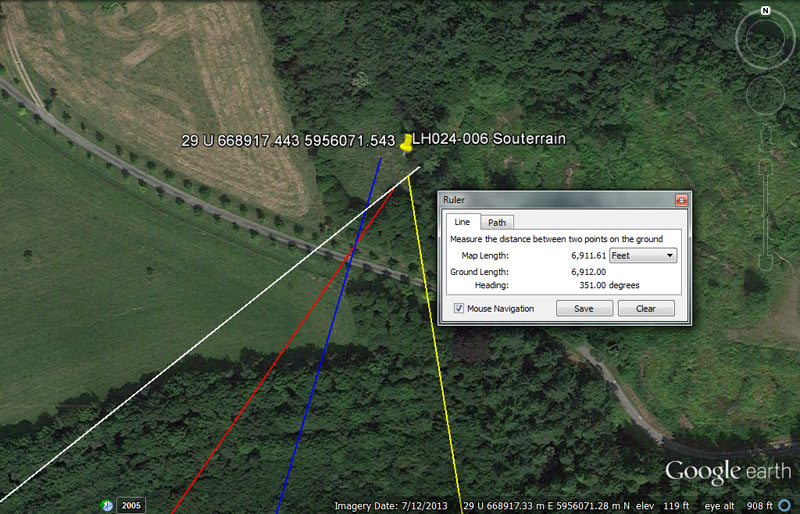More souterrain accommodation was available to orienteers at this spot and the coded distance & angle vectors shown would have been sufficient for orienteers from the 4 main centres to find a cozy spot out of the wind and rain to bed down for the night.

From Knowth = 14400’ @ 51.2-degrees.

This distance is 1-geomancer's mile, an ancient increment found repeatedly to be present between former standing stone circles in Southern England. In the 24883.2-mile equatorial circumference (130636800') the sum of 14400' would be 1/9072nd.
Note: 9072" is the length of the Great Pyramid (756') and 9072' would equate to 1.5 minutes of arc @ 6048' each.

The degree angle is navigational and is in homage to halving quartering, etc., the Earth into smaller divisions (ie) 1/2, 1/4th, 1/8th, 1/16th, 1/32nd, 1/64th. 1/128th, 1/256th, 1/512nd. The sum of 5.12 X 4860' (so-called Roman mile) would be 24883.2-miles.

From Newgrange = 13750’ @ 34.375-degrees.

This distance is 2500 merchant seaman fathoms of 5.5' each and the sum of 13750' X 12 would equate to 10 leagues (3.125 X 5280').

The degree angle is navigational also and the sum of 34.375-miles (34 & 3/8ths) would be half of 1-degree of arc under the 24750-mile (of 5280') assignment.

From Dowth Mound = 8400’ @ 16.2-degrees.

The distance is navigational and equates to 1.25 Irish miles.

The degree angle is in homage to rounded PHI (1.62) and 16.2-miles would be 1/1536th of the equatorial circumference.

From Dowth Henge = 6912’ @ 170.6666-degrees return.

The distance is navigational coding and the sum of 69.12-miles would be 1-degree of arc in the 24883.2 equatorial circumference. This distance would be 1/18900th of the equatorial circumference. The sum of 6048' or 1-minute of arc would be 7/8ths of 6912'.

The degree angle is navigational and 170.6666 (170 & 2/3rds) is simply a 3rd of 256.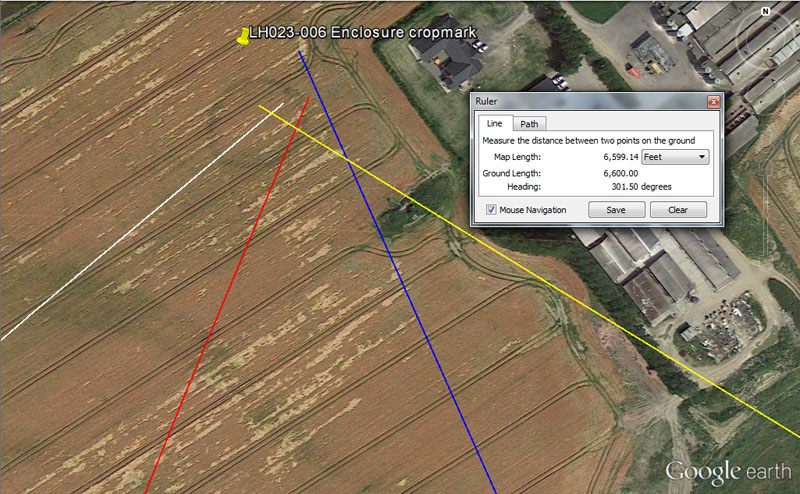Amongst the many types of artificial islands that the wayfaring orienteers had to find, measure and analyse mathematically to extract their codes, were ring-ditch structures, two of which sit in this field at Littlegrange. Sometimes the ring-ditch structures were perfectly circular, with a constant coded diameter. Other times they were oval, as in this case, allowing for two coded diameters 90-degrees opposed. Profound navigational tutorials existed in each diameter measurement and converting these to circumferences read in 360-degrees was essential knowledge that navigators had to learn very thoroughly in order to do positional-plotting at sea.

From Knowth = 8750’ @ 50-degrees.

This distance is 1 & 2/3rds miles of 5250'. The 875 value was related to the calendar also and, at the termination of the slightly-in-error count for the Sabbatical cycle (7-solar years & 7.2 lunar years running concurrently) there would be 8.75 intercalary days to add in order to fully correct the solar year count.

The degree angle is self-explanatory.

From Newgrange = 8640 @ 22.5-degrees.

The sum of 8640-years would be 1/3rd of the 25920-year duration of the Precession of the Equinoxes cycle. The value 864 was multi-use and features copiously in ancient calculations. The sun is 864000-miles wide.

The degree angle is self explanatory and 22.5-degrees is 1/16th of a 360-degree circle.

From Dowth Henge = 6600’ @ 121.5-degrees.

This distance is 10 furlongs or 1.25-miles.

The degree angle is lunar and navigational coding simultaneously and there would be 56 intervals of 121.5-days in the 6804-day lunar nutation cycle or 1215' would equate to 1/4th of a so-called Roman mile.

From Dowth Mound = 5184’ @ 336-degrees.

The 5184 value is highly important to all manner of ancient calculations. The design slope angle of the Great Pyramid was 51.84-degrees. The area that the base of the Great Pyramid covers is 5184 square reeds (of 10.5' each). In the cycle of Precession, 5184-years would be 1/5th of the duration. In navigation at sea, 1-league of travel (16500') would create a circumference of 51840', which is 144' per degree of arc, etc.

The degree angle relates to the diameter of the Earth (7920-miles) of which 3360-miles would be the radius.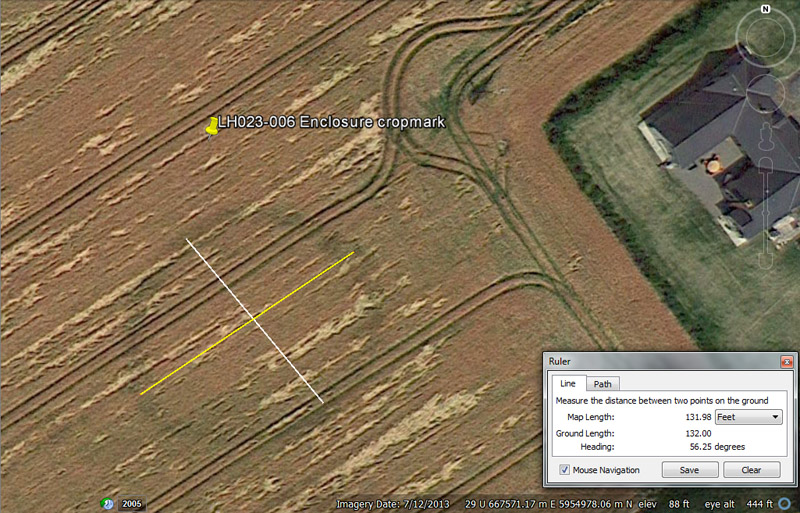This ring-ditch enclosure is now reduced to a crop mark and we may never know the exact, intended cross-measure codes being taught, but can hazard an educated guess. It would appear that the diameter WSW to ENE was 132' @ 236.25-56.25-degrees, whereas a slightly offset from 90-degrees opposed cross-measure would achieve 110' @ 140-320-degrees.

So, here are the tutorials that would have accompanied these cross-measures:

Firstly, the cross-measures offered tutorials in navigation using the "11" family of numbers mile of 5280' and 132' (yellow line) would equate to 1/40th of a mile, whereas 110' would be 1/48th of a mile.

The version of PI used in converting "11" family incremental diameters to circumferences was 1728/550ths or 3.141818182 to 1. Therefore, 132 X 1728/550ths = 414.72, which, in miles, would be 1/60th of a world configured to be 24883.2-miles in equatorial circumference using miles of 5280' (or only 18.8-miles short of the true equatorial circumference of the Earth).

However, if this cross-measure distance was interpreted to be 131.25' (9-inches shorter) then that would be 1/40th of a mile of 5250'. The version of PI used on this mile (divisible by both 6 & 7 simultaneously) would be 22/7ths in order to achieve a perfect circumference reading. Therefore, 131.25' X 22/7ths = 412.5' of circumference. The sum of 412.5-miles would be 1/60th of the 24750-mile equatorial circumference.

From this exercise, we can see that the navigational systems using miles of either 5250' or 5280' were intertwined by PI and were compatible within an integrated system.

The two-way degree angles nominated to express the long cross-measure of this oval ring-ditch are coded also. The angle of 56.25 would be 5/32nds of a 360-degree circle. Alternatively, the angle of 236.25-degrees contains lunar coding and there would be 1.5 X 236.25-days in the lunar year of 354.375-days.

Looking now at the shorter cross-measure (white line), the sum of 110' X 1728/550ths = 345.6' of circumference. The sum of 345.6-miles would be 1/72nd of the 24883.2-mile equatorial circumference. It's of interest that the original design circuit of the Sarsen Circle at Stonehenge was 345.6' or 30 (lintels) X 11.52'.

However, if this cross-measure was interpreted as 109.375' (109 & 3/8ths), then that value would be 1/48th of the 5250' mile. Therefore 109.375 X 22/7ths = 343.75 and this value in miles would be 1/72nd of the 24750-mile equatorial circumference.

The nominated two-way coded angles for this cross-measure run from 140 - 320-degrees. The value 14 was useful within the Sabbatical calendar system, where the solar count was 26 bi-weeks of 14-days, running for 7-years until 8.75 (9) intercalary days were added to correct the calendar shortfall. The value 320 was multi-use and the 360-degree compass was also divided into 320 divisions simultaneously.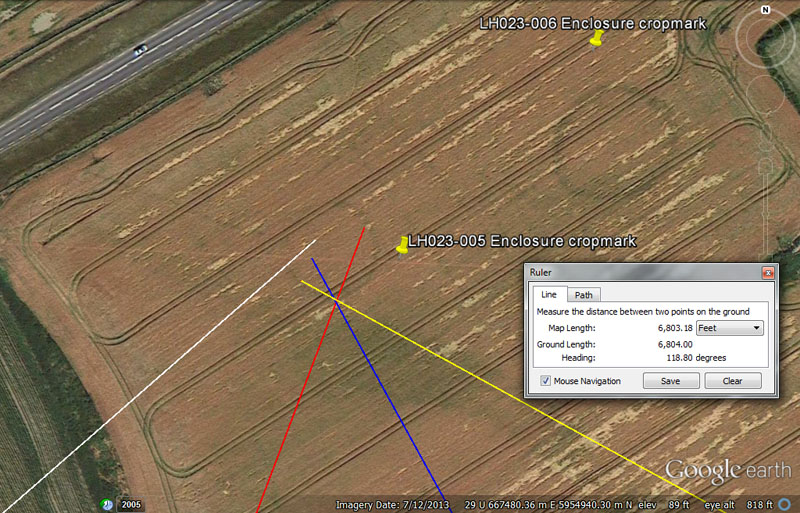Lines run in from the main centres and resolve within this second, smaller crop-mark in the same Littlegrange field.

From Knowth = 8400’ @ 229.166666-degrees.

This distance is 1.25 ancient Irish miles.

The degree angle is navigational and 22.91666-miles (22 & 11/12ths) would be 1/3rd of 1-degree of arc under the 24750-mile navigational method.

From Newgrange = 8400 @ 20.736-degrees.

The distances of both Knowth and Newgrange chambered mounds to this site are equidistant @ 1.25 ancient Irish miles.

The degree angle is navigational coding and the sum of 2073.6-miles would be 1/12th of the 24883.2-mile equatorial circumference. The largest of the 3 Egyptian Royal Cubit types was 20.736".

From Dowth Mound = 5156.25’ @ 151.2-degrees.

This equals 3000 of the mid-range Egyptian Royal Cubit @ 20.625". The sum of 515.625-miles (515 & 5/8ths) would be 1/48th of the 24750-mile circumference.

The degree angle is navigational and 1512' would be 1/4th of 1-minute of equatorial arc (6048') under the system that used 24883.2-miles of 5250'. Alternatively, 1512.5' would be 1-minute of equatorial arc under the navigational system that used 24750-miles of 5280'

From Dowth Henge = 6804’ @ 118.8-degrees.

This distance is in homage to the lunar nutation cycle of 6804-days.

The angle is in homage to the 24750-mile circumference of the Earth and 1/11th of that would be 11880000'.

Continue